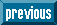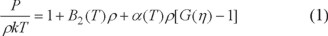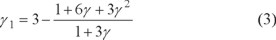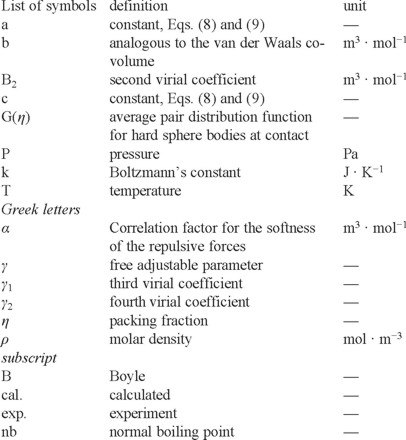## Indicators

•Cited by SciELO
•Access statistics

## Related links

•Similars in SciELO

## Print version ISSN 1870-249X

### J. Mex. Chem. Soc vol.58 n.2 México Apr./Jun. 2014

Article

Song and Mason Equation of State for Refrigerants

Farkhondeh Mozaffari

Department of Chemistry, College of Sciences, Persian Gulf University, Boushehr, Iran. mozaffarif@gmail.com

Received February 10th, 2014
Accepted April 21st, 2014

Abstract

In this work, the Song and Mason equation of state has been applied to calculate the PVT properties of refrigerants. The equation of state is based on the statistical-mechanical perturbation theory of hard convex bodies. The theory has considerable predictive power, since it permits the construction of the PVT surface from the normal boiling temperature and the liquid density at the normal boiling point. The average absolute deviation for the calculated densities of 11 refrigerants is 1.1%.

Key words: equation of state; liquid density; normal boiling point; refrigerants; statistical mechanics.

Resumen

En este trabajo, la ecuación de estado de Song y Mason se ha utilizado para calcular las propiedades PVT de los refrigerantes. La ecuación de estado se basa en la teoría de la perturbación de la mecánica estadística de cuerpos convexos duros. La teoría tiene una gran capacidad de predicción, ya que permite la construcción de la superficie PVT sólo a partir de la temperatura y de la densidad del líquido en el punto de ebullición normal. La desviación absoluta media de las densidades calculadas de 11 refrigerantes es del 1.1%.

Palabras clave: Ecuación de estado; densidad del líquido; punto de ebullición normal; refrigerantes; mecánica estadística.

Introduction

The ozone exhausting properties of chlorofluorocarbons (CFCs) led to restrict the use of such chemical substances. These fluids were primarily used as refrigerants, but are also important as blowing agents in polymer foam manufacture and cleaning solvents in electronic circuit manufacture. An optimum alternative to replace existing chlorofluorocarbons is required to develop accurate thermodynamic information. Due to the difficulties in obtaining the detailed experimental data for all promising substitutes, there is the large demand on the theoretical method.

Recently molecular simulation methods [1-3] have been applied to predict thermophysical properties of refrigerants. Despite the punctuality, molecular simulation methods are computationally expensive and need accurate potential energy function for this purpose.

The description of properties of fluids using accurate equation of state is another method [4,5]. In physics, equation of state attempts to describe the relationship between pressure, temperature and volume for a given substance. Once the equation of state established, all thermodynamic behavior of this substance can be predicted. Most modern theories of liquids have been developed over the past years based on the recognition that the structure of a liquid is determined primarily by repulsive forces, so that fluids of hard bodies can serve as useful reference system and the influence of the attractions and the softness of repulsions are considered as perturbation in statistical-mechanics perturbation theories [6, 7] of fluids. Additionally, attempts have been made to develop new integral equations .

This paper is aimed at elucidating how the equation of state by Song and Mason  can be used with even less input information for refrigerants over a wide range of temperatures and pressures. In particular, knowledge of only two constant, the normal boiling temperature and the liquid density at normal boiling point as scaling parameters is sufficient to predict the thermophysical behavior of refrigerants over a wide range of temperatures and pressures.

Theoretical Equation of State

Song and Mason  proposed an analytical equation of state for convex-molecular fluids based on statistical-mechanical perturbation theory. The equation of state is of the form:where P is the pressure, ρ is the molar (number) density, B2(T) is the second virial coefficient, α(T) is the contribution of the repulsive forces to the second virial coefficient, G(η) is the average pair distribution function at contact for equivalent hard convex bodies, η is the packing fraction, and kT is the thermal energy per one molecule. Song and Mason  adopted the following form for G(η), which is shown to be accurate for hard convex bodies [9, 10].where γ1 and γ2 are chosen to reproduce the correct third and fourth virial coefficients. In practice γ1 and γ2 can be approximated in terms of a single nonsphericity parameter γ, equal to unity for hard spheres. The parameters γ1 and γ2 have been defined in terms of γ as :andThe packing fraction, η, is given bywhere b is the van der Waals covolume and can be defined in terms of α as :Once the intermolecular potential energy function is known, the temperature-dependent parameters B2(T), α(T), and b(T) can be found by three integrations, and γ is the best found by fitting available P-V-T data .

The second virial coefficient B2(T) has a central role in the equation of state, Eq. (1); it is used both directly and as the source of a scaling constant for calculation of α(T) and b(T) . In fact B2(T) is used to determine the Boyle parameters, the Boyle volume and the Boyle temperature, and it is shown that when reduced in terms of the Boyle volume α(T) and b(T) are universal functions of the reduced temperature . This means that one can also use experimental values of B2(T) over a wide range of temperatures to determine the Boyle parameters, and hence α(T) and b(T). But accurate potential energy functions for real fluid to calculation the B2(T) are scarce. Of course, the experimental second virial coefficient is another good source for using the equation of state (EoS). But experimental second virial coefficients have not been derived for all systems over a wide range of temperatures. Therefore, it seems reasonable to find other methods to evaluate the second virial coefficient. The most generally useful method to predict of the volumetric properties of fluids, such as the second virial coefficient, is the use of the hypothesis of corresponding states, which came originally from van der Waals in his well-known EoS.

In the absence of accurate values of the second virial coefficients, there are some corresponding-states methods by which the second virial coefficients and the other two temperature-dependent parameters can be calculated with reasonable accuracy [12-17].

In these methods we need two scaling constants, one to reduce the second virial coefficient and one to reduce the temperature. In the conventional law of corresponding states the critical temperature and the critical volume are used for this purpose, but the critical parameters are either scarce or not measured accurately due to the experimental difficulties at the critical point. Eslami  showed that using two easily measurable scaling constants, the normal boiling temperature and the liquid density at the normal boiling point, one can generate universal plots of the reduced second virial coefficient versus the reduced temperature. In the absence of accurate values of B2(T), we have used the following correlation :where, ρnb and Tnb represent the liquid density at the normal boiling point and the normal boiling temperature, respectively. Then the empirical equation given by Song and Mason  for α/vB and b/vB as a function of T/TB, using Lennard-Jones (12-6) potential, was rescaled by Tnb and ρnb, instead of TB and vB as:andwhereThe remaining problem is to find γ from PVT data. This adjustable parameter is determined by fitting the high density experimental PVT data. Once the value of the constant γ is determined, the entire volumetric behavior is established. The values of γ for each fluid are given in Table 1. When the temperature-dependent parameters together with the values of γ are known, Eq. (1) can be applied to calculate the PVT properties.

Results and discussion

In this work, 11 refrigerants were studied with the Song and Mason equation of state, and our essential result is that this equation (1), together with correlation equations, Equations (7)-(9), to calculate the saturated and compressed liquid and gas density of refrigerants over a wide range of temperatures and pressures. Actually the purpose of this work is to show how this equation of state can be used with even less input information for refrigerants. Two constants are needed for each refrigerant, Tnb and ρnb, which are readily available, and we obtained them from references [18, 19]. Values obtained for γ as well as normal boiling temperature and liquid densities at the boiling point and acentric factors (required for the Peng-Robinson equation of state to be characterized) for 11 refrigerants are given in Table 1. The saturation and compressed liquid densities for these refrigerants were made for many thermodynamic points, and the results are given in Table 2 along with the AAD (average absolute deviation). All of the results in this table are compared with experimental data [20-22]. As Table 2 shows; the agreement between the calculated and experimental data of these refrigerants is very good. The deviations of the Peng-Robinson EoSs results are given in Table 2 as AAD for comparison. Also, this equation of state is applied for vapor density R11, R23, R124 and R134a at different temperature and pressure and the results are compared with experimental data  in Fig. 1. As Fig. 1. shows; the agreement between the calculated vapor densities and the experimental data is very good.Comparison of our calculated densities in Tables 2, Fig. 1. with experiment [20-23] show that the present equation of state can well reproduce the density of refrigerants over a wide range of temperatures and pressures. There is no need to know an accurate potential energy function, or to know the values of the critical constants for the calculation of the second virial coefficients. It can be calculated using simple scaling constants, which are readily available. The other two temperature dependant parameters, α(T) and b(T), are insensitive to the details of the potential energy functions and are just obtainable from the repulsive branch of the potential, or as it is described in this work by knowing just two readily available scaling constants, which are readily available.

The analytic nature of the equation of state also leads to difficulties with the two phase region, because the equation produces van der Waals loops in the subcritical P-V isotherms instead of the correct first-order vapor-liquid transitions.The vapor pressure can, nevertheless, be predicted by application of the Maxwell equal-area construction to the loops. But, the results are not satisfactory.

NomenclatureConclusion

In the present work, only two empirical parameters, normal boiling temperature and liquid densities at the boiling point, which are readily available, are needed to predict the liquid and gas densities of these refrigerants without knowledge of the exact potential energy function or the experimental second virial coefficients.

Acknowledgment

I thank the research Committee of Persian Gulf University.

References

1. Fermeglia, M.; Pricl, S. Fluid Phase Equil. 1999, 158-160, 49-58.         [ Links ]

2. Lisal, M.; Budinsky, R.; Vacek, V.; Aim, K. Int. J. Thermophys. 1999, 20, 163-174.         [ Links ]

3. Vega, C.; Saager, B.; Fischer, J. Molec. Phys. 1989, 68, 1079-1093.         [ Links ]

4. Chapmann, W. G.; Jackson, G.; Gubbins, K. E. Mol. Phys. 1988, 65, 1057-1062.         [ Links ]

5. Boublik, T.; Vega, C.; Diaz-Pena, M. J. Chem. Phys. 1990, 93, 730-736.         [ Links ]

6. Barker, A.; Henderson, D. J. Chem. Phys. 1967, 47, 4714-4721.         [ Links ]

7. Weeks, J. D.; Chandler, D.; Andersen, H. C. J. Chem. Phys. 1971, 54, 5237-5247.         [ Links ]

8. Bretonnet, J. L.; Jakse, N. Phys. Rev. B. 1994, 50, 2880-2888.         [ Links ]

9. Song, Y.; Mason, E. A. Phys. Rev. A. 1990, 42, 4743-4748.         [ Links ]

10. Song, Y.; Mason, E. A. Phys. Rev. A. 1990, 41, 3121-3124.         [ Links ]

11. Song, Y.; Mason, E. A. J. Chem. Phys. 1989, 91, 7840-7853.         [ Links ]

12. Eslami, H. J. Nucl. Matter. 2005, 336, 135-139.         [ Links ]

13. Mehdipour, N.; Boushehri, A. Int. J. Thermophys. 1998, 19, 331-340.         [ Links ]

14. Song, Y.; Mason, E. A. Fluid Phase Equil. 1992, 75, 105-115.         [ Links ]

15. Boushehri, A.; Mason, E. A. Int. J. Thermophys. 1993, 14, 685-697.         [ Links ]

16. Eslami, H. Int. J. Thermophys. 2000, 21, 1123-1137.         [ Links ]

17. Mozaffari, F.; Eslami, H.; Boushehri, A. Int. J. Thermophys. 2007, 28, 1-8.         [ Links ]

18. Sharafi, Z.; Boushehri, A. Int. J. Thermophys. 2005, 26, 785-794.         [ Links ]

19. Maftoon-Azad, L.; Javanmardi, M.; Boushehri, A. Fluid Phase Equil. 2005, 236, 237-240.         [ Links ]

20. Perry, R. H.; Green, D. W. Perry's Chemical Engineers' Handbook, McGrraw-Hill, New York, 1997, 205-308.         [ Links ]

21. Defibaugh, D. R.; Moldover, M. R. J. Chem. Eng. Data. 1997, 42, 160-168.         [ Links ]

22. Ihmels, E. C.; Horstmann, S.; Fischer, K.; Scalabrin, G.; Gmehling, J. Int. J. Thermophys. 2002, 23, 1571-1585.         [ Links ]

23. http://www.refrigerants.com/pdf/2011_Ref%20Guide_print.pdf.         [ Links ]All the contents of this journal, except where otherwise noted, is licensed under a Creative Commons Attribution License